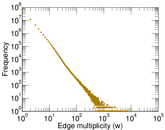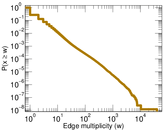# TREC (disks 4–5)

This is the bipartite network of 556,000 text documents from the Text Retrieval Conference's (TREC) Disks 4 and 5, containing 1.1 million words. Each edge represents one document-word inclusion.

 Code `TR` Internal name `gottron-trec` Name TREC (disks 4–5) Data source http://www.nist.gov/tac/data/data_desc.html#TREC AvailabilityDataset is available for download Consistency checkDataset passed all tests Category Text network Node meaning Document, word Edge meaning Inclusion Network formatBipartite, undirected Edge typeUnweighted, multiple edges

## Statistics

 Size n = 1,729,302 Left size n1 = 556,077 Right size n2 = 1,173,225 Volume m = 151,632,178 Unique edge count m̿ = 83,629,405 Wedge count s = 1,604,790,310,718 Claw count z = 58,090,382,597,882,208 Cross count x = 3.122 × 1021 Maximum degree dmax = 457,437 Maximum left degree d1max = 30,701 Maximum right degree d2max = 457,437 Average degree d = 175.368 Average left degree d1 = 272.682 Average right degree d2 = 129.244 Fill p = 0.000 128 187 Average edge multiplicity m̃ = 1.813 14 Size of LCC N = 1,725,011 Diameter δ = 7 50-Percentile effective diameter δ0.5 = 2.953 78 90-Percentile effective diameter δ0.9 = 3.808 95 Median distance δM = 3 Mean distance δm = 3.395 75 Gini coefficient G = 0.854 045 Balanced inequality ratio P = 0.168 945 Left balanced inequality ratio P1 = 0.312 897 Right balanced inequality ratio P2 = 0.033 487 1 Relative edge distribution entropy Her = 0.809 386 Power law exponent γ = 1.503 70 Tail power law exponent γt = 1.401 00 Degree assortativity ρ = −0.068 551 7 Degree assortativity p-value pρ = 0.000 00 Spectral norm α = 44,117.0

## Plots

### Degree distribution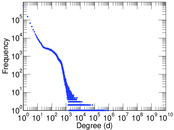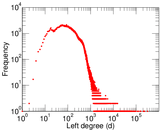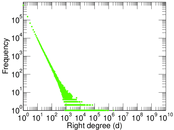### Cumulative degree distribution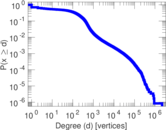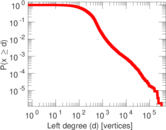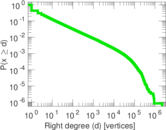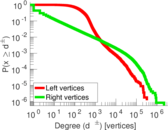### Lorenz curve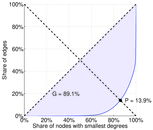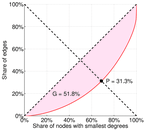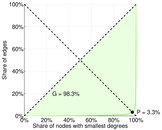### Spectral distribution of the adjacency matrix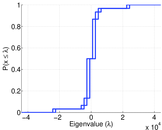### Spectral distribution of the normalized adjacency matrix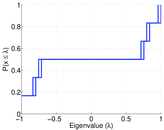### Spectral distribution of the Laplacian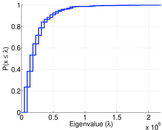### Spectral graph drawing based on the adjacency matrix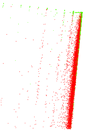### Spectral graph drawing based on the normalized adjacency matrix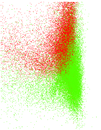### Hop distribution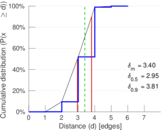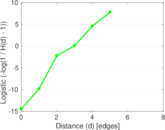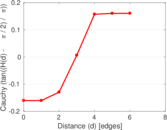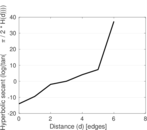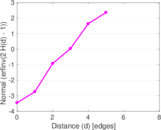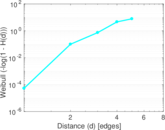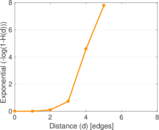### Edge weight/multiplicity distribution